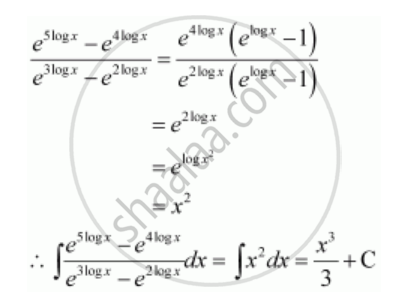Share

# Integrate the Functions (E^(5log X) - E^(4log X))/(E^(3log X) - E^(2log X)) - Mathematics

#### Question

Integrate the functions (e^(5log x) -  e^(4log x))/(e^(3log x) - e^(2log x))

#### SolutionIs there an error in this question or solution?

#### Video TutorialsVIEW ALL 

Solution Integrate the Functions (E^(5log X) - E^(4log X))/(E^(3log X) - E^(2log X)) Concept: Integration as an Inverse Process of Differentiation.
S